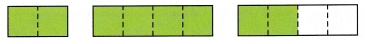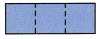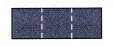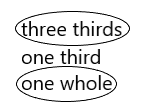# McGraw Hill Math Grade 2 Chapter 7 Lesson 6 Answer Key Describing Whole Rectangles

Practice questions available in McGraw Hill Math Grade 2 Answer Key PDF Chapter 7 Lesson 6 Describing Whole Rectangles will engage students and is a great way of informal assessment.

## McGraw-Hill Math Grade 2 Answer Key Chapter 7 Lesson 6 Describing Whole Rectangles

Describe

Question 1.
Circle the shape that shows four fourths.Explanation:
I drew a circle around the shape that shows four fourths that is 4 parts
So, i drew a circle around the second shape.

Question 2.
How many thirds in the whole rectangle? Write the number.____________ thirds = one whole
3 thirds = one whole

Explanation:
The whole rectangle above is 3 thirds that is one whole.

Question 3.
How many halves in the whole rectangle? Write the number._________ halves = one whole
2 halves = one whole

Explanation:
The whole rectangle above is 2 halves that is one whole.

Question 4.
How much of the rectangle is purple? Circle two answers.three thirds
one third
one whole